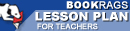Lesson Plans

# Journey Through Genius: The Great Theorems of Mathematics Quiz | Eight Week Quiz E

This set of Lesson Plans consists of approximately 142 pages of tests, essay questions, lessons, and other teaching materials.
 View a FREE sampleName: _________________________ Period: ___________________

This quiz consists of 5 multiple choice and 5 short answer questions through The Bernoullis and the Harmonic Series.

## Multiple Choice Questions

1. Who was Eratosthanes?
(a) He was a teacher and philosopher.
(b) He was a mathematician, and leading doctor.
(c) He was the first to study political sciences.
(d) He was the chief librarian, and a mathematician.

2. Who was the author of the book Elements?
(a) Hippocrates.
(b) Lindemann.
(c) Einstein.
(d) Euclid.

3. Who acted as the gate keepers of knowledge?
(a) Roman emporers.
(b) Greek tradesman.
(c) Greek philosophers.
(d) Arabian scholars.

4. Who, in modern day, is given credit for the calculus method?
(a) Newton,
(b) Johann Bernoulli.
(c) Leibniz.
(d) Both Newton and Leibniz.

5. Which of the following did Dunham concentrate on as one of Newton's great advances?
(a) Quadratic equation.
(b) Quintic theorem.
(c) Area of a sphere.
(d) Binomial theorem.

## Short Answer Questions

1. What did Plato use his inspiration from Euclid for?

2. What did the Pythagorean Theorem accomplish for mathematics?

3. Which of the following was an important proposition given by Euclid's number theory?

4. Which phrase best describes Newton as a student at Cambridge?

5. What did Archimedes manage to prove using Euclid's ideas?

(see the answer key)

 This section contains 323 words (approx. 2 pages at 300 words per page) View a FREE sampleCopyrightsJourney Through Genius: The Great Theorems of Mathematics from BookRags. (c)2019 BookRags, Inc. All rights reserved.
Follow Us on Facebook